floor_floor函数 - CSDN

• 1、floor函数：朝负无穷大方向取整 2、用法说明： y = floor(x) 函数将x中元素取整，值y为不大于本身的最大整数。对于复数，分别对实部和虚部取整 3、用法举例 >> x = [3+4i 6-7i 9+11i 1-4i 3.4-4.5i...
1、floor函数：朝负无穷大方向取整

2、用法说明：

y = floor(x) 函数将x中元素取整，值y为不大于本身的最大整数。对于复数，分别对实部和虚部取整

3、用法举例

>> x = [3+4i 6-7i 9+11i 1-4i 3.4-4.5i 90.67-123i]

x =

1.0e+02 *

Columns 1 through 5

0.0300 + 0.0400i   0.0600 - 0.0700i   0.0900 + 0.1100i   0.0100 - 0.0400i   0.0340 - 0.0450i

Column 6

0.9067 - 1.2300i

>> y = floor(x)

y =

1.0e+02 *

Columns 1 through 5

0.0300 + 0.0400i   0.0600 - 0.0700i   0.0900 + 0.1100i   0.0100 - 0.0400i   0.0300 - 0.0500i

Column 6

0.9000 - 1.2300i

4、附录

>> help floor
floor  Round towards minus infinity.
floor(X) rounds the elements of X to the nearest integers
towards minus infinity.

codistributed/floor
gpuArray/floor

Reference page in Help browser
doc floor


展开全文matlab floor 函数 基本函数
• ## floor() 函数

千次阅读 2019-03-23 11:13:20
查看更多 https://www.yuque.com/docs/share/2a8366d1-bdc5-4faf-95ed-655beea8d8f9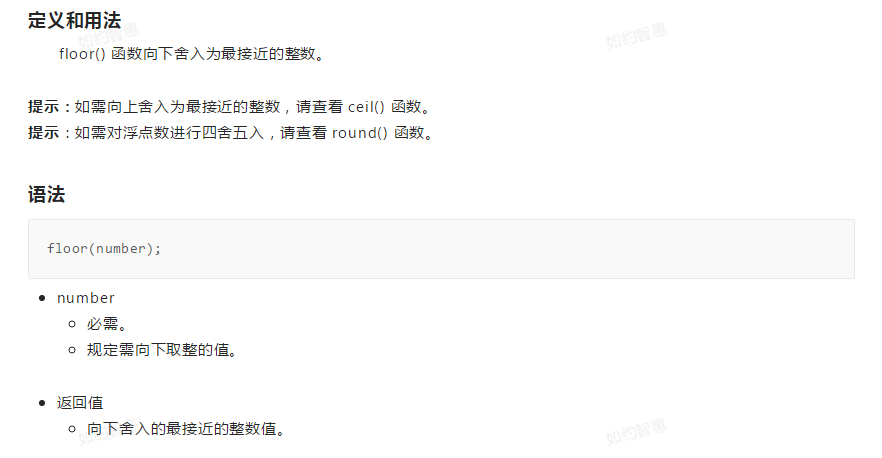查看更多

https://www.yuque.com/docs/share/2a8366d1-bdc5-4faf-95ed-655beea8d8f9

展开全文PHP Math
• 1.floor函数 原型：int 示例 #include&amp;amp;amp;lt;stdio.h&amp;amp;amp;gt; #include&amp;amp;amp;lt;stdlib.h&amp;amp;amp;gt; #include&amp;amp;amp;lt;math.h&amp;amp;amp;gt;...
C语言中

1.floor函数

功能：把一个小数向下取整
即就是如果数是2.2 ，那向下取整的结果就为2.000000
原型：double floor(doube x);
参数解释：
x:是需要计算的数
返回值：
成功：返回一个double类型的数，此数默认有6位小数
无失败的返回值
头文件：#include<math.h>


示例
floor函数计算后的结果为double类型的：

#include<stdio.h>
#include<stdlib.h>
#include<math.h>
int main()
{
double i = floor(2.2);
double j = floor(-2.2);
printf("The floor of 2.2 is %f\n", i);
printf("The floor of 2.2 is %f\n", j);
system("pause");
return 0;
}

运行结果：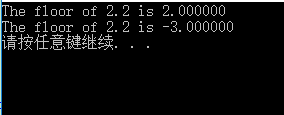floor函数把转换后的结果强转为int类型的：

#include<stdio.h>
#include<stdlib.h>
#include<math.h>
int main()
{
int i = floor(2.2);
int j = floor(2.7);
printf("i=%d,j=%d\n", i, j);
system("pause");
return 0;
}

运行结果：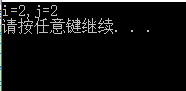ps：把计算结果强转为int后，会丢失精度

2.ceil函数

功能：把一个小数向上取整
即就是如果数是2.2 ，那向上取整的结果就为3.000000
原型：double ceil(doube x);
参数解释：
x:是需要计算的数
返回值：
成功：返回一个double类型的数，此数默认有6位小数
无失败的返回值
头文件：#include<math.h>


示例
ceil函数计算的结果为double类型的：

#include<stdio.h>
#include<stdlib.h>
#include<math.h>
int main()
{
double i = ceil(2.2);
double j = ceil(-2.2);
printf("The ceil of 2.2 is %f\n", i);
printf("The ceil of 2.2 is %f\n", j);
system("pause");
return 0;
}

运行结果：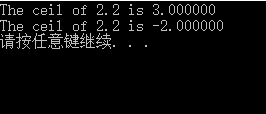ceil函数把计算后的结果强转为int类型的：

#include<stdio.h>
#include<stdlib.h>
#include<math.h>
int main()
{
int i = ceil(2.2);
int j = ceil(2.7);
printf("i=%d,j=%d\n", i, j);
system("pause");
return 0;
}

运行结果：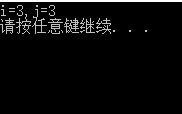3.round函数

功能：把一个小数四舍五入
即就是如果数是2.2 ，那四舍五入的结果就为2
如果数是2.5,那结果就是3
原型：double round(doube x);
参数解释：
x:是需要计算的数
头文件：#include<math.h>


示例

round函数的计算结果为double类型的：

#include<stdio.h>
#include<stdlib.h>
#include<math.h>
int main()
{
double i = round(2.2);
double x = round(2.7);
double j = round (-2.2);
double y = round(-2.7);
printf("The round of 2.2 is %f\n", i);
printf("The round of 2.7 is %f\n", x);
printf("The round of -2.2 is %f\n", j);
printf("The round of -2.7 is %f\n", y);
system("pause");
return 0;
}


运行结果：C++中

1.floor函数

#include<iostream>
using namespace std;
int main()
{
double i = floor(2.2);
double j = floor(-2.2);
cout << "The floor of 2.2 is " << i << endl;
cout << "The floor of -2.2 is " << j << endl;
system("pause");
return 0;
}

运行结果：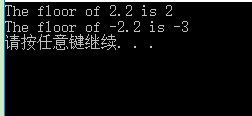2.ceil函数

#include<iostream>
using namespace std;
int main()
{
double i = ceil(2.2);
double j = ceil(-2.2);
cout << "The ceil of 2.2 is " << i << endl;
cout << "The ceil of -2.2 is " << j << endl;
system("pause");
return 0;
}


运行结果：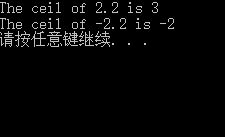3.round函数

#include<iostream>
using namespace std;
int main()
{
double i = round(2.2);
double x = round(2.7);
double j = round(-2.2);
double y = round(-2.7);
cout << "The round of 2.2 is " << i << endl;
cout << "The round of 2.7 is " << x << endl;
cout << "The round of -2.2 is " << j << endl;
cout << "The round of -2.7 is " << y << endl;
system("pause");
return 0;
}

运行结果：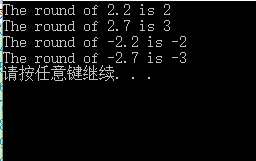展开全文round floor ceil
• floor() 函数 头文件是<math.h> 向下取整，floor(x)获得不大于x参数且最靠近参数x的整数，x参数可以是整数（正负）和浮点数（正负）。
floor() 函数

头文件是<math.h>
向下取整，floor(x)获得不大于x参数且最靠近参数x的整数，x参数可以是整数（正负）和浮点数（正负）。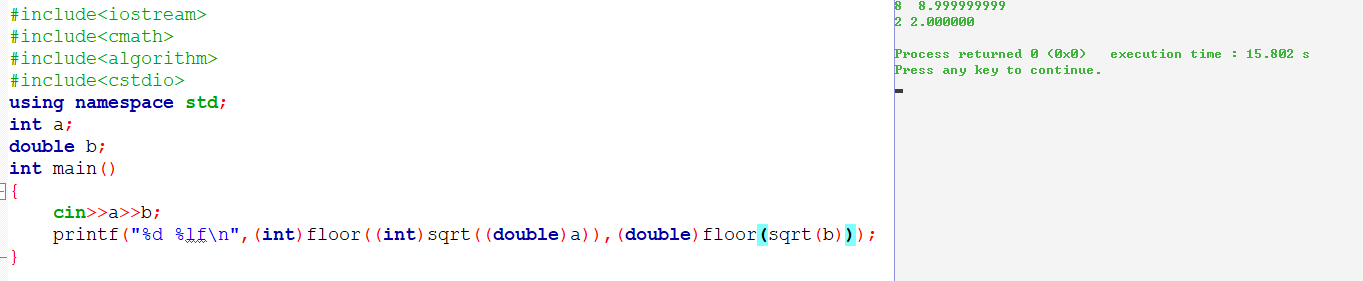展开全文• 2.1 2.6 -2.1 -2.6 floor : 不大于自变量的最大整数 2 2 -3 -3 ceil :不小于自变量的最大整数 3 3c++
•C语言
• 除法：传统除法、floor和真除法 有三种类型的除法操作符，其中一种操作符在python 3.0 中有了变化 x/y: 传统除法和真除法。在python2.7之前，这个操作对于整数会省去小数部分，对于浮点数会保持小数部分 在...
• ## floor函数用法

千次阅读 2019-07-14 19:48:13
floor(x)，也写做Floor(x)，其功能是“向下取整”，或者说“向下舍入”，即取不大于x的最大整数（与“四舍五入”不同，下取整是直接取按照数轴上最接近要求值的左边值，即不大于要求值的最大的那个整数值）。...
• 对一个数进行下舍入。 console.log(Math.ceil(0.60)) // 0 console.log(Math.ceil(0.40)) // 0 console.log(Math.ceil(5)) // 5 console.log(Math.ceil(5.1)) // 5 console.log(Math.ceil(-5.1)) // -6 ...
• ## floor()报错注入

千次阅读 2018-06-11 12:07:45
floor()报错注入准确地说应该是floor,count,group by冲突报错是当这三个函数在特定情况一起使用产生的错误。首先看经典的floor注入语句:and select 1 from (select count(*),concat(database(),floor(rand(0)*2))x ...sql注入学习笔记 floor
• ## 取整函数（ceil、floor、round）

万次阅读 多人点赞 2016-12-06 10:20:35
取整函数主要有三种：ceil()、floor()、round()先来说说ceil()函数ceil：在英文中，是天花板的意思，有向上的意思，所以，此函数是向上取整，它返回的是大于或等于函数参数,并且与之最接近的整数，在这里以java为例...java ceil floor round 取整函数
• ## MATLAB中floor、round、ceil、fix区别

万次阅读 多人点赞 2016-09-20 10:45:24
Matlab取整函数有: fix, floor, ceil, round.具体应用方法如下： fix朝零方向取整，如fix(-1.3)=-1; fix(1.3)=1; floor，顾名思义，就是地板，所以是取比它小的整数，即朝负无穷方向取整，如floor(-1.3)=-2; floor...
• ## C++ floor函数

万次阅读 2011-02-11 15:14:00
的确，用C++也算挺久了，竟然不知道C++库中的floor函数，可以轻易实现这个功能，唉，感慨~~~~   就不多说别的了，下面说一下关于floor函数: 1、函数原型： double floor ( double x );...c++ float 语言 c
• ## Java Math的 floor,round和ceil的总结

万次阅读 多人点赞 2014-10-21 15:23:52
floor 返回不大于的最大整数 round 则是4舍5入的计算，入的时候是到大于它的整数（当-1.5时可见，四舍五入后得到的结果不是我们期待的，解决办法是先对他取绝对值，然后在用round方法）round方法，它表示“四舍五入...math java string class 算法
• ## oracle ceil和floor函数

万次阅读 2013-01-03 22:50:48
ceil和floor函数在一些业务数据的时候，有时还是很有用的。 ceil(n) 取大于等于数值n的最小整数； floor(n)取小于等于数值n的最大整数 如下例子 SQL> select ceil(9.5) from dual;    CEIL(9.5) ----------...oracle Oracle ORACLE
• ## floor函数 与 ceil函数

万次阅读 多人点赞 2011-08-22 10:29:03
floor函数 　floor(x),有时候也写做Floor(x)，其功能是“下取整”，或者说“向下舍入”，即取不大于x的最大整数   (与 “四 舍 五入”不同，下取整是直接去掉小数部分)，例如： 　x=3.14，floor语言 c
• ## 向下取整floor函数

万次阅读 2012-08-08 16:17:28
floor函数 　floor(x),有时候也写做Floor(x)，其功能是“下取整”，或者说“向下舍入”，即取不大于x的最大整数(与“四舍五入”不同，下取整是直接去掉小数部分)，例如： 　x=3.14，floor(x)=3 　y...语言 c
• ## MATLAB Floor 用法

万次阅读 2017-11-06 10:14:06
1、floor函数：朝负无穷大方向取整 2、用法说明：  y = floor(x) 函数将x中元素取整，值y为不大于本身的最小整数。对于复数，分别对实部和虚部取整 3、用法举例 >> a= 2*rand(4) a =  1....
• ## C++中的floor()函数

千次阅读 2017-03-24 20:00:05
y = floor(x)。 则y等于：不大于x的最大整数。详细参考：http://www.cplusplus.com/reference/cmath/floor/C++ floor
• Floor(n) 作用 返回小于等于n的最大整数 例子 SELECT FLOOR(15.1) FROM dual;FLOOR(15.1) ----------- 15SELECT FLOOR(-15.1) FROM dual;FLOOR(-15.1) ------------SELECT FLOOR(0.1) FROM dual;FLOOR(0.1) -----...oracle floor round trunc functions
...